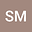New fixed point results in extended $b-$metric-like spaces via simulation functions with applications
•The main ambition proposed in this article is to provide new fixed point results for triangular $\alpha-$orbital admissible contractions via some auxiliary and simulation functions in the frame of extended $b-$metric-like spaces. As an application, we prove the existence of a unique solution for a nonlinear fractional differential equation with exponential weighted integral boundary conditions via the generalized proportional fractional derivative of Caputo type with order $\beta\in (n-1, n]$. Further, we demonstrate the usability of our results through several examples.